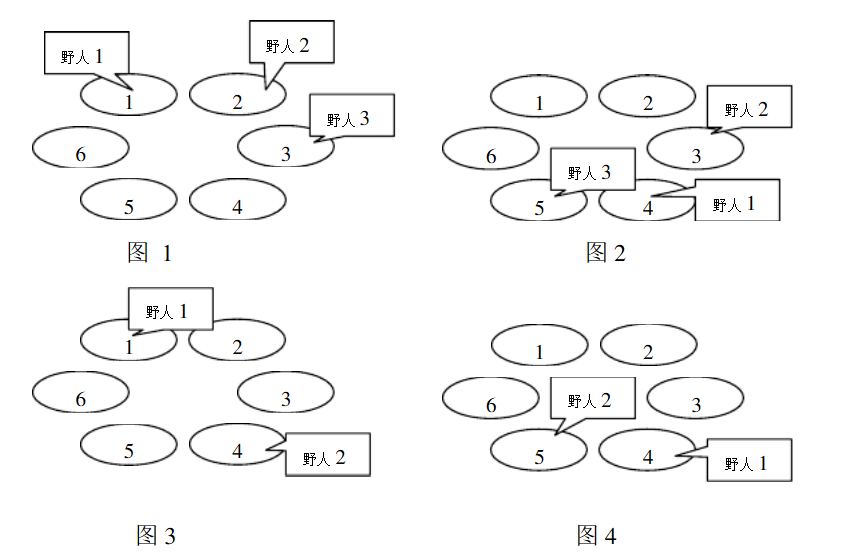# NOI2002 荒岛野人

### 题目

Luogu P2421### 分析

[C_i+kP_i equiv C_j+kP_j pmod{M} ]

[k(P_i-P_j) equiv C_j-C_i pmod{M} ]

(M) 已知，那我们可以用EXGCD求出上面的同余方程的一个解，进而求出最小正整数解，若最小正整数解 (k leq min(L_i,L_j)) 则会发生山洞重合的情况，野人会发生冲突，否则不会

### 代码

#include<bits/stdc++.h> #define ll long long using namespace std;  int n; int c, p, l;  ll gcd(ll a, ll b) {     return a % b == 0 ? b : gcd(b, a % b); }  ll exgcd(ll a, ll b, ll & x, ll & y) {     if(b == 0) {         x = 1;         y = 0;         return a;     }     ll d = exgcd(b, a % b, y, x);     y -= a / b * x;     return d; }  bool check(int m) {     for(int i = 1; i <= n; i++) {         for(int j = i + 1; j <= n; j++) {             int a = ((p[j] - p[i]) % m + m) % m;             int b = ((c[i] - c[j]) % m + m) % m;             ll d = gcd(a, m);             if(b % d != 0)                 continue;             ll x, y, t;             exgcd(a, m, x, y);             t = m / d;             x = (x * b / d % t + t) % t;             if(x <= min(l[i], l[j]))                 return false;         }     }     return true; }  int main() {     cin >> n;     int minn = n;     for(int i = 1; i <= n; i++) {         cin >> c[i] >> p[i] >> l[i];         minn = max(minn, c[i]);         c[i]--;     }     for(int m = minn; m <= 1000000; m++) {         if(check(m)) {             cout << m << endl;             break;         }     }     return 0; } 

### 推荐这些技术文章：

[NOI2002] 荒岛野人

$c_1+p_1s\equiv c_2+p_2s\pmod{k}$变形可得：
$(p_1-p_2)s-rk=c_2-c_1$可以发现这就是一个 $$a=p_... 【Coel.解题报告】【推柿子的基本思路】[NOI2002] 荒岛野人 题前碎语 还有13天就要期考了，可我还是在机房颓题目。 其实我本来不是很想颓的，可是这道题对于学习扩欧来说很重要，所以还是放进来一下。 题目简介 洛谷传送门 题目描述 克里特岛以野人群居而著称。岛上有排列成环行的 \(m$$ 个山洞。这些山洞顺时针编号为 $$1,2,\dots ,m$$ 。岛上住着 $$n$$ 个野人，一开始依次住在山洞 $$C_1,C_2,\dots ,C_n$$中，以后每年...

NOI 2002 荒岛野人

#include<bits/stdc++.h>
using namespace std;
#define lowbit(x) x&(-x)
#define ll long long
int T;
ll gcd(ll aa,ll bb){
if(bb)return gcd(bb,aa%bb);
return aa;
}
int main(...

2019-2020 XX Open Cup, Grand Prix of Korea

2019-2020 XX Open Cup, Grand Prix of Korea

​ 1427314831a：过了A
​ Ryker0923 ：过了G，提供了A的思路

1）后期乏力

Educational Codeforces Round 129(补题)

C. Double Sort

C++
// Problem: C. Doubl...

Educational Codeforces Round 129 (Rated for Div. 2) A - D 题解

A. Game with Cards

#include <iostream>
#include <cstdio>
#include <algorithm>
#include <vector>
#include <string>
#include <queue>
#i...

【2022neuoj刷题打卡】2581~2590

2581

//Author:Fczhao
//Language:cpp
#include <bits/stdc++.h>
using namespace std;
signed main(){
#ifdef FCZHAO
freopen("1.in", "r", stdin);
freope...

#include <iostream>
using std::cin; using std::cout; using std::endl;

#include <vector>
using std::vector;

#include <algorith...

UVA1589 象棋 Xiangqi

THE END